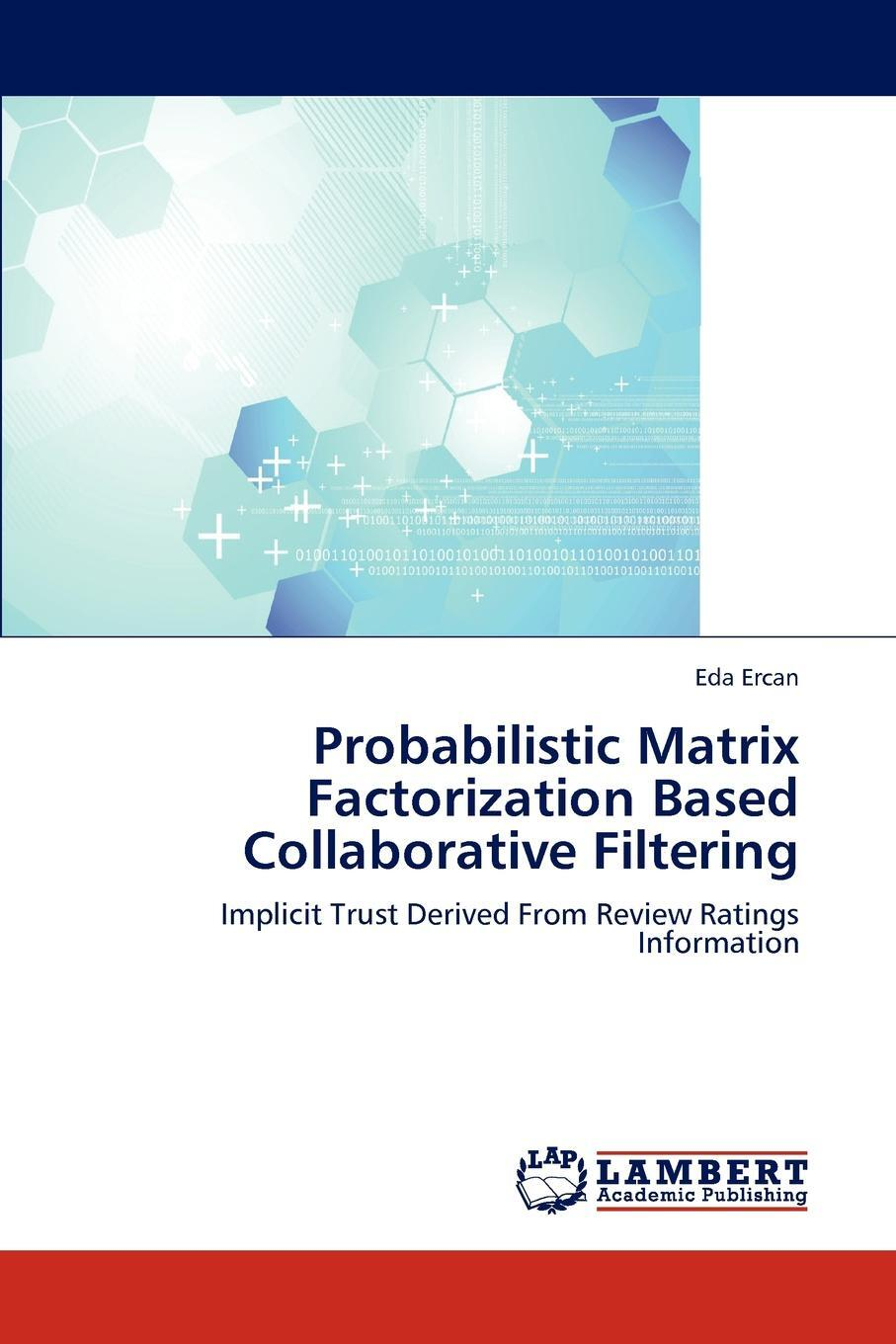# Book: Eda Ercan «Probabilistic Matrix Factorization Based Collaborative Filtering»Recommender systems aim to suggest relevant items that are likely to be of interest to the users using a variety of information resources such as user profiles, trust information and users past predictions. However, typical recommender systems suffer from poor scalability, generating incomprehensible and not useful recommendations and data sparsity problem.In this work, we have proposed a probabilistic matrix factorization based local trust boosted recommendation system which handles data sparsity, scalability and understandability problems. The method utilizes the implicit trust in the review ratings of users. The experiments conducted on Epinions.com dataset showed that our method compares favorably with the methods in the literature.In the scope of this work, we have analyzed the effect of latent vector initialization in matrix factorization models; different techniques are compared with the selected evaluation criteria.

Категории:

Формат: 152x220, 108 стр.

ISBN: 9783846597545

Универсальный код товара: 9783846597545

### Характеристики

 Вес: (г) 184 Ширина упаковки: (мм) 152 Высота упаковки: (мм) 6 Глубина упаковки: (мм) 229 Обложка: Мягкая обложка Размеры: 22.900/15.200/0.600 Вес: 0.184 Возраст: 12

Цена: 8789 руб

### Look at other dictionaries:

• Non-negative matrix factorization — NMF redirects here. For the bridge convention, see new minor forcing. Non negative matrix factorization (NMF) is a group of algorithms in multivariate analysis and linear algebra where a matrix, , is factorized into (usually) two matrices, and… …   Wikipedia

• Probabilistic latent semantic analysis — (PLSA), also known as probabilistic latent semantic indexing (PLSI, especially in information retrieval circles) is a statistical technique for the analysis of two mode and co occurrence data. PLSA evolved from Latent semantic analysis, adding a… …   Wikipedia

• Document-term matrix — A document term matrix or term document matrix is a mathematical matrix that describes the frequency of terms that occur in a collection of documents. In a document term matrix, rows correspond to documents in the collection and columns… …   Wikipedia

• Integer factorization — In number theory, integer factorization is the way of breaking down a composite number into smaller non trivial divisors, which when multiplied together equal the original integer.When the numbers are very large, no efficient integer… …   Wikipedia

• List of statistics topics — Please add any Wikipedia articles related to statistics that are not already on this list.The Related changes link in the margin of this page (below search) leads to a list of the most recent changes to the articles listed below. To see the most… …   Wikipedia

• Latent class model — In statistics, a latent class model (LCM) relates a set of observed discrete multivariate variables to a set of latent variables. It is a type of latent variable model. It is called a latent class model because the latent variable is discrete. A… …   Wikipedia

• Latent Dirichlet allocation — In statistics, latent Dirichlet allocation (LDA) is a generative model that allows sets of observations to be explained by unobserved groups which explain why some parts of the data are similar. For example, if observations are words collected… …   Wikipedia

• algebra — /al jeuh breuh/, n. 1. the branch of mathematics that deals with general statements of relations, utilizing letters and other symbols to represent specific sets of numbers, values, vectors, etc., in the description of such relations. 2. any of… …   Universalium

• Tikhonov regularization — Tikhonov regularization, named for Andrey Tikhonov, is the most commonly used method of regularization of ill posed problems. In statistics, the method is known as ridge regression, and, with multiple independent discoveries, it is also variously …   Wikipedia

• List of mathematics articles (P) — NOTOC P P = NP problem P adic analysis P adic number P adic order P compact group P group P² irreducible P Laplacian P matrix P rep P value P vector P y method Pacific Journal of Mathematics Package merge algorithm Packed storage matrix Packing… …   Wikipedia

• Kalman filter — Roles of the variables in the Kalman filter. (Larger image here) In statistics, the Kalman filter is a mathematical method named after Rudolf E. Kálmán. Its purpose is to use measurements observed over time, containing noise (random variations)… …   Wikipedia